﻿
Reach Us+44-1474-556909
A New Number Theory: Considerations about the (3-n)d Algebra

Journal of Applied & Computational MathematicsOpen Access

All submissions of the EM system will be redirected to Online Manuscript Submission System. Authors are requested to submit articles directly to Online Manuscript Submission System of respective journal.

A New Number Theory: Considerations about the (3-n)d Algebra

Sonaglioni L*
Free Professionist, Italy
*Corresponding Author: Sonaglioni L, Free Professionist, Italy, Tel: 388-0579470, Email: [email protected]

Received Date: Nov 19, 2018 / Accepted Date: Nov 24, 2018 / Published Date: Nov 30, 2018

Abstract

Keywords: Quaternions; Operator theory; Algebra; Tensor methods

Introduction

About 3 years ago, we have seen that we can build a commutative (3-n)d algebra [1-3] quite similar to the standard 2d complex algebra; open arguments are lacks about derivatives and integrals, this algebra is not distributive. What can we do with this algebra?

The non distributive property does not permit and easy analytical exam of a problem (in general), but this is not a limitation if the approach is an applied computational study .

For the (3-n)d algebra, in general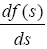does not exist, also a (3-n) d integral ∫ f(s).ds depends on the path, that means it couldn’t calculate a primitive of f(s) (note that f(s).ds is a (3-n)d product [3,4].

A point in the 3d space  can always be written as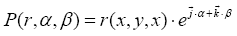(1)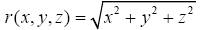The operator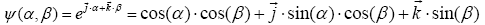(2)

can be seen as a 3d number function that plots a surface sphere of radius 1 around the origin, while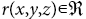is a standard real function and represent the modulus of the vector identified by the tern (x,y,z).

It’s quite simple to demonstrate that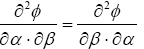So the ψ(α,β) operator is differentiable.

In an applied computational approach, given an algebraic definition of a f(s) (as for example a polynomial function), we can always study the function in polar notation or in algebraic notation: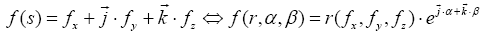and, because the ψ(α,β) operator is differentiable, also f(s) is always differentiable (if r(fx,fy,fz) is differentiable).

This mean we can always calculate the gradient of a (3-n)d function, the divergence of a (3-n)d function, the rotor of a 3d function, etc. and in general we can calculate partial derivatives of a generic multi dimensions f(s) along any direction.

About the integrals, the main question is: what is the goal?

If the goal is to calculate a volume or a surface , there are no problems.

We can always calculate the elementary volume or elementary surface along any directions of the operator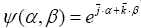, because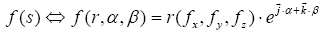to estimate dr by starting from any point in the 3d space along any direction. To estimate the elementary cube by changing the coordinates (see the function Project_A_on_B(..) in the 3d-4d appendix code [2,3] or the elementary surface.

As for example, let us consider a generic surface, a R2→R3 transformation: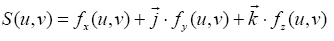The area of the surface is: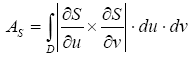(3)

Where D is the domain of (u,v)

The integral in eqn. (3) can be easily computed in the same way as it have been with the standard 3d algebra in a convenient symbolic form.

For more dimension (4 and more), ψ(α,β) is still differentiable, and the consideration of above are the same; of course, in this case, the elementary volume is a hyper-elementary volume, the elementary surface is a hyper-elementary surface, and so on.

The operator ψ(α, β,…) can be seen as a 4d (or more dimensions) operator that plots a hyper surface sphere of radius 1 around the 4d (or more dimensions) origin space.

A last consideration: we are talking about a 3-n (d) commutative algebra, where the 3-n (d) sum and product are well defined (it is well defined the algebraic product, ), and where we have seen that the 3-n (d) functions are always differentiable and the computational integrals are always possible.

For these reasons I suppose it is possible to define the discrete and the standard Fourier and Laplace transformations for a 3-n (d) time function, but I really can’t it imagine if this can be, in concrete, useful or not.

Conclusions

It introduced the basis of a new multi dimension commutative algebra that may be good extension of the standard 2d complex algebra.

This algebra permit to treat multi dimensions problems exactly in the same way we use to do actually with a simplified symbolic form, and with the extension of the definition of the product as number product; also the extensions of the multi dimensions external and internal product, comply with the standard operators used in the standard 3d problems.

For multi dimensions numeric problems, they are also well defined the extended concepts of the sum and product, the exponential function (the ψ (α ,β ,..) operator) and the log function.

References

Citation: Sonaglioni L (2018) A New Number Theory: Considerations about the (3-n)d Algebra. J Appl Computat Math 7: 427. DOI: 10.4172/2168-9679.1000427

Copyright: © 2018 Sonaglioni L. This is an open-access article distributed under the terms of the Creative Commons Attribution License, which permits unrestricted use, distribution, and reproduction in any medium, provided the original author and source are credited.

Select your language of interest to view the total content in your interested language

Recommended Journals
Viewmore
Article Usage
• Total views: 419
• [From(publication date): 0-0 - Oct 16, 2019]
• Breakdown by view type
• HTML page views: 381Can't read the image? click here to refresh